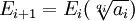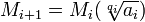## Wednesday, October 22, 2008

### Abel's Proof: Field Extensions: Step 1: Proof

The content in today's blog is taken from the essay by Michael I. Rosen entitled "Niels Hendrik Abel and Equations of the Fifth Degree."

In today's blog, I present the key lemma that I will use to establish Step 1 of the proof using field extensions. For the proof using the ideas that Niels Abel originally presented, see here.

Lemma 1:

Let:where qi is prime, ai ∈ Ei

and Ei, Ei+1 are fields that include the roots of unity

L is a field such that (Ei ∩ L) ⊂ (Ei+1 ∩ L) ⊂ L and includes the roots of unity.

Mi+1 = Ei+1 ∩ L

Mi = Ei ∩ L

γ ∈ Mi+1 but not in Mi

Then:

There exist:

γi = c0 + c1ζi-1β + c2ζ2(i-1)β2 + ... + cq-1ζ(q-1)(i-1)βq-1

such that:

ci ∈ Ei

β ∈ Ei+1, βq ∈ Ei

γi ∈ Mi+1

ζ is a primitive qth root of unity

Proof:

(1) From our assumptions, we know that γq ∈ Mi

(2) Let P(x) be a polynomial such that P(γ) = 0, that is, γ is a root for P(x).

(3) We further know that (see Corollary 3.1, here) there exists an element β ∈ Ei+1 such that βq ∈ Ei

and there exists b0, b2, ..., bq-1 ∈ Ei such that:

γ = b0 + β + b2β2 + ... + bq-1βq-1

(4) We can define a function Q(γ) such that Q(γ) = P(b0 + x + b2x2 + ... + bq-1xq-1)

(5) From this definition, it is clear that Q(β) = P(γ) = 0 so that β is a root for Q(γ).

(6) Let a = βq

(7) It is clear that β is the root of Yq - a.

(8) We also know that Yq - a is irreducible over Mi [See Lemma 1, here]

(9) But since β is a root for both Yq - a and Q(Y), it follows that Yq - a divides Q(Y) and every root of Yq - a is also a root of Q(Y). [See Theorem 3, here]

(10) By the Fundamental Theorem of Algebra [See Theorem, here], we know that there are q roots for Yq - a and they are: &beta, ζβ, ζ2β, ..., ζq-1β where ζ is a primitive qth root of unity [see here for review of the primitive roots of unity].

(11) Since Yq - a divides Q(Y) and ζiβ is a root of Yq - a, it follows that Q(ζiβ) = 0 for all integers i.

(12) So, the numbers [see step #5 above]:

γ = γ1 = b0 + β + b2β2 + ... + bq-1βq-1

γ2 = b0 + ζβ + b2ζ2β2 + ... + bq-1ζq-1βq-1

...

γq = b0 + ζq-1β + b2ζ2(q-1)β2 + ... + bq-1ζ(q-1)(q-1)βq-1

are all roots of P(x). That is, P(γk) = 0 for k = 1, ..., q.

(13) We also know that the numbers γ1, γ2, ..., γq are all in L since:

(a) γ ∈ Mi+1 = Ei+1 ∩ L [from step #1 above]

(b) So, γ ∈ Ei+1 and γ ∈ L.

(c) Then, using Lemma 5, here, we can see that each γk ∈ L.

QED

Lemma 2:

Let:where qi is prime, ai ∈ Ei

and Ei, Ei+1 are fields that include the roots of unity

L is a field such that (Ei ∩ L) ⊂ (Ei+1 ∩ L) ⊂ L and includes the roots of unity.

Mi+1 = Ei+1 ∩ L

Mi = Ei ∩ L

Then:where qi is prime, ai ∈ Mi

Proof:

(1) Let y be an element such that y ∈ Mi+1 but y is not an element in Mi

We know that there is at least one such element since Mi ⊂ Mi+1

(2) Using Lemma 1 above, we know that there exists y1, ..., yq such that each yi ∈ Mi+1 such that:

y = y1 = b0 + β + b2β2 + ... + bq-1βq-1

y2 = b0 + ζβ + b2ζ2β2 + ... + bq-1ζq-1βq-1

...

yq = b0 + ζq-1β + b2ζ2(q-1)β2 + ... + bq-1ζ(q-1)(q-1)βq-1

where β ∈ Ei+1 but not in Ei

βq ∈ Ei

b0, ..., bq-1 ∈ Ei

(3) Now, if we multiply each equation in step #13 above by ζ1-i, we get:

y = y1 = b0 + β + b2β2 + ... + bq-1βq-1

ζ-1y2 = ζq-1y2 = ζq-1b0 + ζ0β + b2ζ1β2 + ... + bq-1ζq-2βq-1

...

ζ1-qyq = ζ1 = ζb0 + ζ0β + b2ζ2(q-1)+1β2 + ... + bq-1ζ(q-1)(q-1)+1βq-1

(4) If we add up each row, then we have the following values for each column [Using Lemma 4, here]:

b0 + ζ-1b0 + ... + ζ1-qb0 = b0(1 + ζ1*(q-1) + ζ2*(q-1) + ... + ζ(q-1)*(q-1)) = b0*0 = 0. [Since q doesn't divide q-1.]

β + ζ0β + ... + ζ0β = qβ

b2β2 + ζ1b2β2 + ... + ζq-1b2β2 = b2β2(1 + ζ1*1 + ζ2*1 + ... + ζ(q-1)*1) = b2β2*0 = 0

...

bq-1βq-1 + ζq-2bq-1βq-1 + ... + ζ2b2β2 = bq-1βq-1(1 + ζ1*1 + ζ2*1 + ... + ζ(q-1)*1) = bq-1βq-1*0 = 0

(5) So, adding each of the columns together gives us the following equation:

β = (1/q)∑ (i=1,q) ζ1-iyi ∈ L

We know that β ∈ L since all ζ1-i,q are in L (by the given) and yi ∈ L by step #2 above.

(6) Thus β ∈ L ∩ Ei+1 = Mi+1 and βq = b ∈ L ∩ E = Mi

(7) Let γ ∈ Mi+1 but not Mi

(8) Then there exist (see Lemma 1 above using the same value β as before):

γi = c0 + c1ζi-1β + c2ζ2(i-1)β2 + ... + cq-1ζ(q-1)(i-1)βq-1 with ci∈ Ei and each γi ∈ Mi+1

(9) If we multiply each γi by ζk(1-i) and add up each column in the same way that we did in steps #15 - #17), we get:

ckβk = ∑ (i=1,q) ζk(1-i)γi .

(10) Now since ζk(1-i), γi ∈ Mi+1, it follows that each ckβk ∈ Mi+1

(11) Since β ∈ Mi+1 [See step #6 above], it follows that:

βk ∈ Mi+1, and using step #10 above, we get ck ∈ Mi+1

(12) But if ck ∈ Mi+1 = Ei+1 ∩ L, it follows that ck ∈ L.

(13) And since ck ∈ Ei [see step #8 above] and ck ∈ L [see step #12 above], it follows that ck ∈ Mi = Ei ∩ L.

(14) Thus, we have show that:where qi is prime, ai ∈ Mi

QED

Theorem 2:

If E/K is a radical tower and L ⊆ E, then it follows that L/K is also a radical tower.

Proof:

(1) Since E/K is a radical tower, we have (see Definition 4, here):

K = E0 ⊂ E1 ⊂ E2 ⊂ ... ⊂ Em = E

where for each Ei we have:such that: qi is prime, ai ∈ Ei

(2) Since K ⊂ L, it follows that:

K = E0 ∩ L

(3) Further, since Ei ⊂ Ei+1, it follows that:

Ei ∩ L ⊆ Ei+1 ∩ L

(4) Since L ⊆ E, it follows that L = E ∩ L

(5) Putting steps #2, #3, and #4 together gives us:

K = (E0 ∩ L) ⊆ (E1 ∩ L) ⊆ ... ⊆ (Em-1 ∩ L) ⊆ L

(6) Now, if remove all cases where Ei ∩ L = Ei+1 ∩ L and renumber, then we get the following:

K = (E0 ∩ L) ⊂ (E1 ∩ L) ⊂ ... ⊂ En = L

(7) Using Lemma 2 above, we know that for each Ei, (Ei+1 ∩ L)/(Ei ∩ L) is a field extension.

(8) Thus, we have shown that L/K is tower of radicals.

QED

References